This is the sixth post of a project on collaborative filtering based on the MovieLens 100K dataset. The remainder of this post is straight out of a Jupyter Notebook file you can download here. You can also see it here on GitHub.

Now that I've implemented 3 main classes of collaborative filtering methods (similarity-based, alternating least squares (ALS), and stochastic gradient descent (SGD)), it's time to see how they stack up to each other.

To compare models, I'll use 2 different metrics: mean absolute error (MAE) and normalized discounted cumulative gain (NDCG). MAE measures about how many stars off all the predictions are on average. This is useful information, but in most recommendation situations, the user will only see a few of the top recommendations given to them. The NDCG score tells us how "good" the top few recommendations are, with decreasing weight given the farther you go down the list.

Usually, NDCG will be reported for a certain number of recommendations. If we just care about the first 3 recommendations, we would computer NDCG@3. If there were no movies that the user would have rated more highly than these 3, then NDCG@3 is 1.0. Lower values mean other movies would have gotten higher ratings.

If you're interested, the math looks like this:

Given a vector $\mathbf{r}$ of $k$ recommendations from most to least recommended, discounted cumulative gain (DCG) is given by:

$$DCG@k = \sum_{i=1}^k \frac{r_i}{\log_2(i+1)}$$

Normalized DCG (NDCG) is just DCG divided by the maximum possible DCG:

$$NDCG@k = \frac{DCG@k}{\max_{\mathbf{r}} DCG@k}$$
In :
Expand Code
In :
Expand Code

# 2. Load the Data¶

Let's load and examine the ratings data. If you're following along (i.e. actually running these notebooks) you'll need to make sure to run the first one to download the data before running this one.

In :
Expand Code
First 5:

userId movieId rating timestamp
214 259 255 4 1997-09-19 23:05:10
83965 259 286 4 1997-09-19 23:05:27
43027 259 298 4 1997-09-19 23:05:54
21396 259 185 4 1997-09-19 23:06:21
82655 259 173 4 1997-09-19 23:07:23
Last 5:

userId movieId rating timestamp
46773 729 689 4 1998-04-22 19:10:38
73008 729 313 3 1998-04-22 19:10:38
46574 729 328 3 1998-04-22 19:10:38
64312 729 748 4 1998-04-22 19:10:38
79208 729 272 4 1998-04-22 19:10:38
In :
Expand Code
In :
Expand Code
Fold 0:
Train: between 13 and 491 movies per user
Test:  between 7 and 246 movies per user
Fold 1:
Train: between 13 and 491 movies per user
Test:  between 7 and 246 movies per user
Fold 2:
Train: between 14 and 492 movies per user
Test:  between 6 and 245 movies per user

In :
Expand Code
In :
Expand Code

# 3. Choose the best user-based model¶

Let's use cross-validation to examine MAE and NDCG@3 on out-of-sample data and choose the "best" user-based model.

In :
Expand Code
k=1, i_fold=0: MAE=0.8335820137299328, NDCG=0.8419218314194908
k=2, i_fold=0: MAE=0.8055319020030114, NDCG=0.8488402325053938
k=5, i_fold=0: MAE=0.7898301104752179, NDCG=0.8568816660263787
k=10, i_fold=0: MAE=0.7844811512641147, NDCG=0.866351545900177
k=20, i_fold=0: MAE=0.7825949168981733, NDCG=0.8663123680140652
k=50, i_fold=0: MAE=0.7823864170624978, NDCG=0.86451390079912
k=100, i_fold=0: MAE=0.7837336683942548, NDCG=0.864783101784657
k=1, i_fold=1: MAE=0.8103195965310307, NDCG=0.8342529646792158
k=2, i_fold=1: MAE=0.7771523904087133, NDCG=0.8445940280960973
k=5, i_fold=1: MAE=0.7541189079102684, NDCG=0.8544306736447582
k=10, i_fold=1: MAE=0.7472360724615519, NDCG=0.8587829551463168
k=20, i_fold=1: MAE=0.744198914317921, NDCG=0.8616782748602941
k=50, i_fold=1: MAE=0.7440653472763141, NDCG=0.8672427206403276
k=100, i_fold=1: MAE=0.7457825084494136, NDCG=0.8646034532713005
k=1, i_fold=2: MAE=0.8591514716745691, NDCG=0.8349623209950944
k=2, i_fold=2: MAE=0.8341265278764367, NDCG=0.8457560801890412
k=5, i_fold=2: MAE=0.8179387558067737, NDCG=0.85340710020124
k=10, i_fold=2: MAE=0.8132244297036613, NDCG=0.8603510473151312
k=20, i_fold=2: MAE=0.8109264482099519, NDCG=0.8639371740798584
k=50, i_fold=2: MAE=0.8108590433573192, NDCG=0.862968089610793
k=100, i_fold=2: MAE=0.8125660602709356, NDCG=0.8604898319974263

In :
Expand Code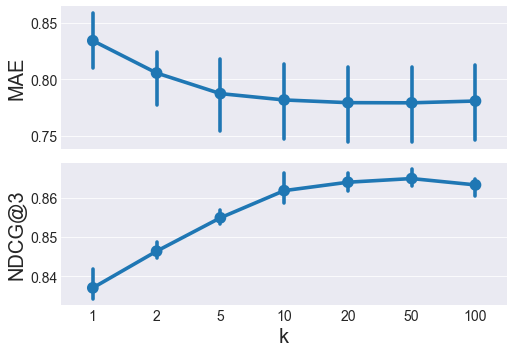NDCG@3 peaks at $k=50$, and MAE is pretty similar between $k=20$ to $100$, so $k=50$ is the winner.

In :
baseline_algo = DampedUserMovieBaselineModel(damping_factor=10)
best_user_model = KNNRecommender(mode='user', k=50, baseline_algo=baseline_algo)


# 4. Choose the best item-based model¶

Let's use cross-validation to examine MAE and NDCG@3 on out-of-sample data and choose the "best" item-based model.

In :
Expand Code
i_fold=0: k=1, k=2, k=5, k=10, k=20, k=50, k=100,
i_fold=1: k=1, k=2, k=5, k=10, k=20, k=50, k=100,
i_fold=2: k=1, k=2, k=5, k=10, k=20, k=50, k=100,

In :
Expand Code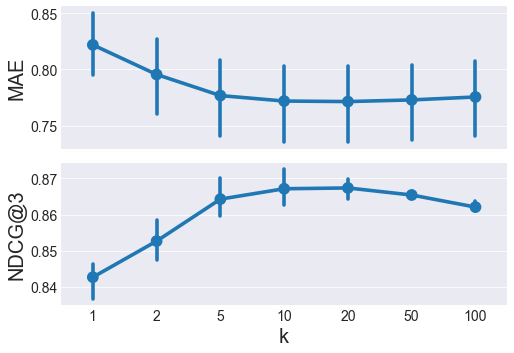Here, $k=10$ and $k=20$ have similar MAE and NDCG@3, we'll favor higher $k$ in nearest neigbor methods because higher $k$ is less prone to overfitting. $k=20$ is the winner of the item-based models.

In :
baseline_algo = DampedUserMovieBaselineModel(damping_factor=10)
best_item_model = KNNRecommender(mode='item', k=20, baseline_algo=baseline_algo)


# 5. Choose the best ALS model¶

Let's use cross-validation to examine MAE and NDCG@3 on out-of-sample data and choose the "best" ALS model.

In :
Expand Code
i_fold=0, k=5: i_epoch=0, 1, 2, 3, 4, 5, 6, 7, 8, 9, 10, 11, 12, 13, 14,
i_fold=0, k=10: i_epoch=0, 1, 2, 3, 4, 5, 6, 7, 8, 9, 10, 11, 12, 13, 14,
i_fold=0, k=50: i_epoch=0, 1, 2, 3, 4, 5, 6, 7, 8, 9, 10, 11, 12, 13, 14,
i_fold=1, k=5: i_epoch=0, 1, 2, 3, 4, 5, 6, 7, 8, 9, 10, 11, 12, 13, 14,
i_fold=1, k=10: i_epoch=0, 1, 2, 3, 4, 5, 6, 7, 8, 9, 10, 11, 12, 13, 14,
i_fold=1, k=50: i_epoch=0, 1, 2, 3, 4, 5, 6, 7, 8, 9, 10, 11, 12, 13, 14,
i_fold=2, k=5: i_epoch=0, 1, 2, 3, 4, 5, 6, 7, 8, 9, 10, 11, 12, 13, 14,
i_fold=2, k=10: i_epoch=0, 1, 2, 3, 4, 5, 6, 7, 8, 9, 10, 11, 12, 13, 14,
i_fold=2, k=50: i_epoch=0, 1, 2, 3, 4, 5, 6, 7, 8, 9, 10, 11, 12, 13, 14,

In :
Expand Code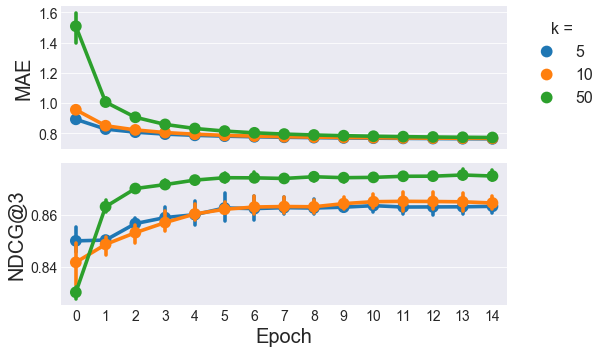In :
Expand Code
i_fold=0, k=20.0: lmbda=0.05
i_fold=0, k=20.0: lmbda=0.1
i_fold=0, k=20.0: lmbda=0.2
i_fold=0, k=50.0: lmbda=0.05
i_fold=0, k=50.0: lmbda=0.1
i_fold=0, k=50.0: lmbda=0.2
i_fold=0, k=100.0: lmbda=0.05
i_fold=0, k=100.0: lmbda=0.1
i_fold=0, k=100.0: lmbda=0.2
i_fold=0, k=200.0: lmbda=0.05
i_fold=0, k=200.0: lmbda=0.1
i_fold=0, k=200.0: lmbda=0.2
i_fold=1, k=20.0: lmbda=0.05
i_fold=1, k=20.0: lmbda=0.1
i_fold=1, k=20.0: lmbda=0.2
i_fold=1, k=50.0: lmbda=0.05
i_fold=1, k=50.0: lmbda=0.1
i_fold=1, k=50.0: lmbda=0.2
i_fold=1, k=100.0: lmbda=0.05
i_fold=1, k=100.0: lmbda=0.1
i_fold=1, k=100.0: lmbda=0.2
i_fold=1, k=200.0: lmbda=0.05
i_fold=1, k=200.0: lmbda=0.1
i_fold=1, k=200.0: lmbda=0.2
i_fold=2, k=20.0: lmbda=0.05
i_fold=2, k=20.0: lmbda=0.1
i_fold=2, k=20.0: lmbda=0.2
i_fold=2, k=50.0: lmbda=0.05
i_fold=2, k=50.0: lmbda=0.1
i_fold=2, k=50.0: lmbda=0.2
i_fold=2, k=100.0: lmbda=0.05
i_fold=2, k=100.0: lmbda=0.1
i_fold=2, k=100.0: lmbda=0.2
i_fold=2, k=200.0: lmbda=0.05
i_fold=2, k=200.0: lmbda=0.1
i_fold=2, k=200.0: lmbda=0.2

In :
Expand Code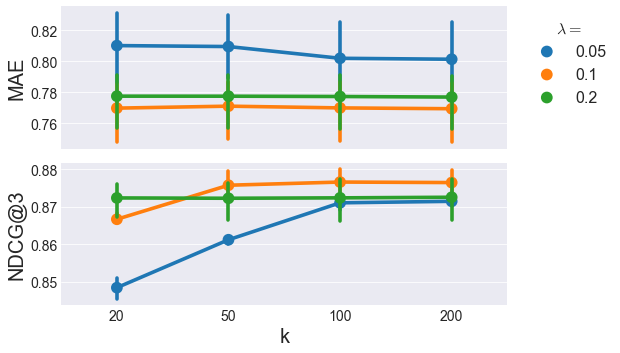Here, it looks like MAE is pretty flat with respect to the learning rate $\lambda$, but NDCG@3 shows some interesting variations. The highest NDCG@3 comes from $\lambda=0.1$ and $k>=50$. With matrix factorization methods like ALS, we want to favor lower $k$ for better generalizability, so $\lambda=0.1$ and $k=50$ is the winner of the ALS category.

In :
baseline_algo = DampedUserMovieBaselineModel(damping_factor=10)
best_als_model = ALSRecommender(k=50, lmbda=0.1, max_epochs=15, baseline_algo=baseline_algo)


# 6. Choose the best SGD model¶

Let's use cross-validation to examine MAE and NDCG@3 on out-of-sample data and choose the "best" SGD model.

In :
Expand Code
i_fold=0, k=5: i_epoch=0, 1, 2, 3, 4, 5, 6, 7, 8, 9, 10, 11, 12, 13, 14,
i_fold=0, k=10: i_epoch=0, 1, 2, 3, 4, 5, 6, 7, 8, 9, 10, 11, 12, 13, 14,
i_fold=0, k=50: i_epoch=0, 1, 2, 3, 4, 5, 6, 7, 8, 9, 10, 11, 12, 13, 14,
i_fold=1, k=5: i_epoch=0, 1, 2, 3, 4, 5, 6, 7, 8, 9, 10, 11, 12, 13, 14,
i_fold=1, k=10: i_epoch=0, 1, 2, 3, 4, 5, 6, 7, 8, 9, 10, 11, 12, 13, 14,
i_fold=1, k=50: i_epoch=0, 1, 2, 3, 4, 5, 6, 7, 8, 9, 10, 11, 12, 13, 14,
i_fold=2, k=5: i_epoch=0, 1, 2, 3, 4, 5, 6, 7, 8, 9, 10, 11, 12, 13, 14,
i_fold=2, k=10: i_epoch=0, 1, 2, 3, 4, 5, 6, 7, 8, 9, 10, 11, 12, 13, 14,
i_fold=2, k=50: i_epoch=0, 1, 2, 3, 4, 5, 6, 7, 8, 9, 10, 11, 12, 13, 14,

In :
Expand Code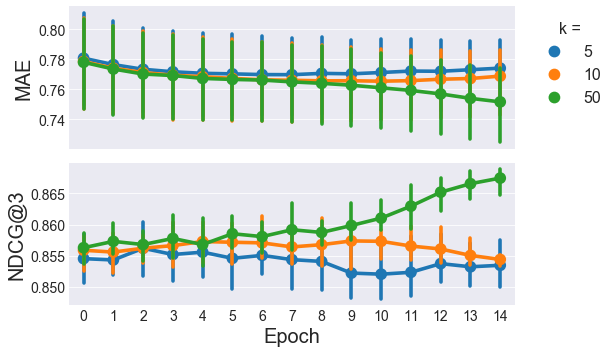In :
Expand Code
i_fold=0, learning_rate=0.001, reg=0.0
i_fold=0, learning_rate=0.001, reg=0.001
i_fold=0, learning_rate=0.001, reg=0.01
i_fold=0, learning_rate=0.01, reg=0.0
i_fold=0, learning_rate=0.01, reg=0.001
i_fold=0, learning_rate=0.01, reg=0.01
i_fold=1, learning_rate=0.001, reg=0.0
i_fold=1, learning_rate=0.001, reg=0.001
i_fold=1, learning_rate=0.001, reg=0.01
i_fold=1, learning_rate=0.01, reg=0.0
i_fold=1, learning_rate=0.01, reg=0.001
i_fold=1, learning_rate=0.01, reg=0.01
i_fold=2, learning_rate=0.001, reg=0.0
i_fold=2, learning_rate=0.001, reg=0.001
i_fold=2, learning_rate=0.001, reg=0.01
i_fold=2, learning_rate=0.01, reg=0.0
i_fold=2, learning_rate=0.01, reg=0.001
i_fold=2, learning_rate=0.01, reg=0.01

In :
Expand Code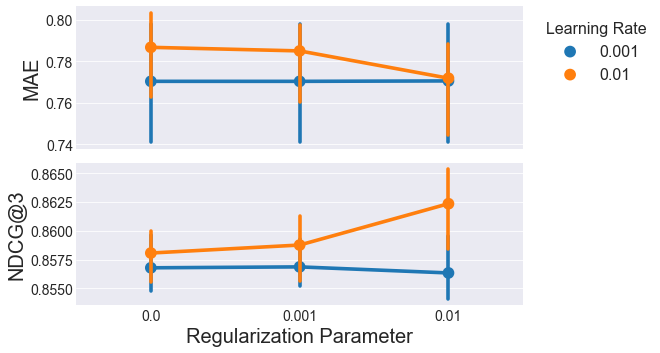In :
reg = 0.01
best_sgd_model = SGDRecommender(k=50, learning_rate=0.01, max_epochs=30, damping_factor=10,
user_reg=reg, item_reg=reg, user_bias_reg=reg, item_bias_reg=reg)


# 7. Let's compare the top methods of each category.¶

In :
Expand Code
i_fold=0, model=user
i_fold=1, model=user
i_fold=2, model=user
i_fold=0, model=item
i_fold=1, model=item
i_fold=2, model=item
i_fold=0, model=als
i_fold=1, model=als
i_fold=2, model=als
i_fold=0, model=sgd
i_fold=1, model=sgd
i_fold=2, model=sgd

In :
Expand Code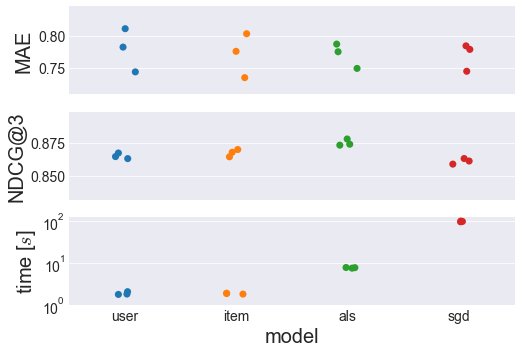There's a lot of information in the 3 charts above. The charts show 3 different metrics (Mean Absolute Error, Normalized Discounted Cumulative Gain, and time) for the best user-based, item-based, ALS, and SGD models I found. Each metric/model combination has 3 points, representing the values for each of the 3 folds used for cross-validation.

The MAE doesn't seem to change much across the different models, although the variance seems to be slightly smaller for the matrix factorization methods (ALS and SGD) compared to the nearest neighbors methods (user-based and item-based).

The NDCG@3 does seem to vary across the different models though, with the highest score going to the ALS model. NDCG@3 is arguably the more useful metric for a recommender system, so as long as very high speeds aren't important, ALS wins here.

If this ALS model is too slow for a particular application, the item-based method would be the next choice. Both user- and item-based recommenders have similarly fast training speeds, with item-based having a slightly higher NDCG@3 score. The slower execution of the ALS and SGD models are likely related to the number of iterations over for loops required in each iteration.

As they are right now, my user- and item-based models don't need any python for loops during training. ALS has $n_{users} + n_{movies}$ python for loop iterations per epoch, and SGD has $n_{ratings}$ iterations per epoch, which is about an order of magnitude higher. I specify "python" for loops, because the vectorized operations used in user-based, item-based, and ALS models have for loops in c which are much faster than those in python. By optimizing code with something like cython or numba, I could certainly drop the training time for ALS and SGD.

If you want to play with these models interactively, check out my recommender notebook. With this notebook, you could choose whichever user you want, show some of their favorite movies, then display the top recommendations given by any of these 4 models.